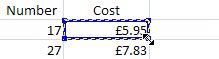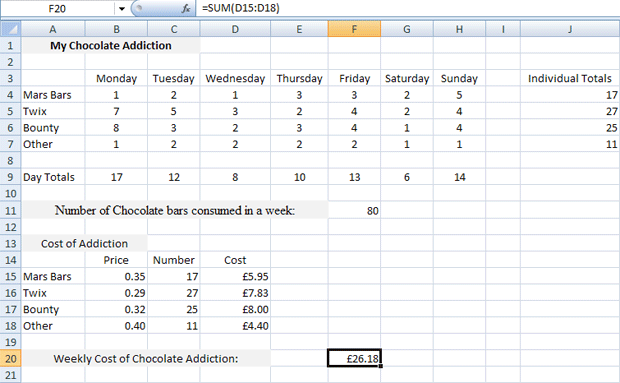To finish off the Excel spreadsheet you have been working on in this section, we'll add figures for the weekly cost and yearly costs of the chocolate addiction. We'll use AutoFill and SUM.

The bottom of our spreadsheet looks like this:We now have how much each individual chocolate bar is costing us each week. The next things to do is to add them all up to arrive at a weekly figure for all chocolate bars.

To calculate the weekly cost of the chocolate addiction, you can use the Excel SUM function. But there's an even easier way - use Auto Fill and SUM. Try this.

• Click inside cell F20
• Click inside the Formula bar at the top and enter = SU
• When you see the drop down list of functions, double click SUM
• Now click inside D15 of you spreadsheet
• Excel will enter the Cell for you in the formula bar:• Notice the marching ants around Cell D15, and that there is a blue border with blue squares
• Hold your mouse over the bottom right blue square until your cursor changes to a double-headed arrow:• Now hold your left mouse button down and drag down to cell D18
• Let go and Excel will enter the rest of the formula for you:Press the enter key on your keyboard to finish off the rest of the formula:If you did that correctly, you should have a figure of 26.18 in cell F20. That's how much our chocolate bar addiction is costing each week.

To work out how much the addiction is costing every year, we can multiply the weekly cost of the addiction by 52 (the number of weeks in a year). First, enter some suitable text in cell A21, something like "Annual Cost of Chocolate addiction". The answer can then go in cell F21, under the weekly cost.

• Then click into the formula bar at the top
• Enter the following:

= F20 * 52

Hit the enter key on your keyboard, and the correct answer should appear.

Cell F20 is where the weekly total is. Excel already knows what formula is inside of this cell, so only the cell reference is needed. After the multiply symbol, we then only need to enter the number of weeks in a year.

The answer you should have in cell F21 is 1, 361.36. You spreadsheet should look like ours below:The formula we just used mixes a cell reference with a number. Excel doesn't mind you doing it this way, just as long as there's something to multiply. So you can do things this way:

= 26.18 * 52

Or this way:

= F20 * 52

If you have the number 52 typed into say cell H20, you could just do this:

= F20 * H20

Whichever way you choose, though, just remember to use the asterisk to multiply things.

In the next tutorial, you'll learn how to add a Comment to a Cell in Excel.

<--Back to the Excel Contents Page